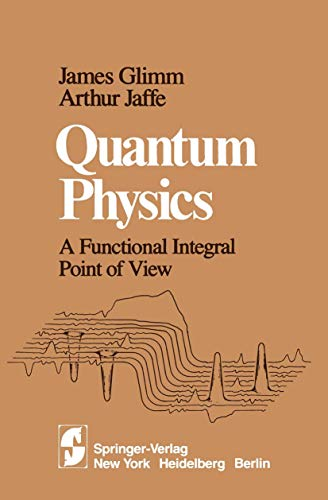# Quantum Physics: A Functional Integral Point of View

## Glimm, J.; Jaffe, A.

Valutazione media 2
( su 1 valutazioni fornite da Goodreads )Vedi tutte le copie di questo ISBN:

This book is addressed to one problem and to three audiences. The problem is the mathematical structure of modem physics: statistical physics, quantum mechanics, and quantum fields. The unity of mathemati� cal structure for problems of diverse origin in physics should be no surprise. For classical physics it is provided, for example, by a common mathematical formalism based on the wave equation and Laplace's equation. The unity transcends mathematical structure and encompasses basic phenomena as well. Thus particle physicists, nuclear physicists, and con� densed matter physicists have considered similar scientific problems from complementary points of view. The mathematical structure presented here can be described in various terms: partial differential equations in an infinite number of independent variables, linear operators on infinite dimensional spaces, or probability theory and analysis over function spaces. This mathematical structure of quantization is a generalization of the theory of partial differential equa� tions, very much as the latter generalizes the theory of ordinary differential equations. Our central theme is the quantization of a nonlinear partial differential equation and the physics of systems with an infinite number of degrees of freedom. Mathematicians, theoretical physicists, and specialists in mathematical physics are the three audiences to which the book is addressed. Each of the three parts is written with a different scientific perspective.

Le informazioni nella sezione "Riassunto" possono far riferimento a edizioni diverse di questo titolo.

Product Description:

Book by None

Le informazioni nella sezione "Su questo libro" possono far riferimento a edizioni diverse di questo titolo.

### Altre edizioni note dello stesso titolo

#### Edizione in evidenza

ISBN 10: 0387964770 ISBN 13: 9780387964775
Casa editrice: Springer-Verlag, 1987
BrossuraSpring..., 1987
RilegatoSpringer, 1981
BrossuraSpring..., 1987
Rilegato## 1.Quantum Physics: A Functional Integral Point of View

Editore: Springer
ISBN 10: 0387905510 ISBN 13: 9780387905518
Nuovo Quantit�: 1
Da
RushLtd
(Denver, CO, U.S.A.)

Descrizione libro Springer. Condizione: new. Codice articolo think0387905510

Compra nuovo
EUR 147,34
Convertire valuta
Spese di spedizione: GRATIS
In U.S.A.
Destinazione, tempi e costi